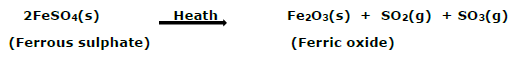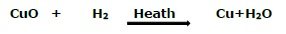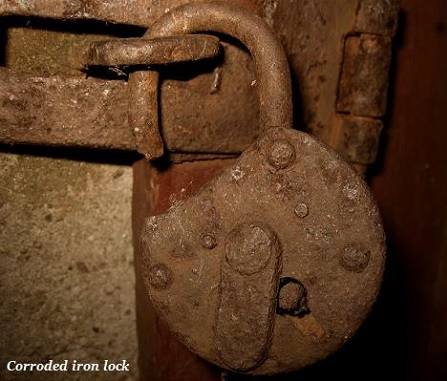# Chemistry - Chemical Reactions & Equations

## Introduction

• A process in which one or more chemical substances react with other chemical substance and converted to one or more different substances is known as chemical reaction.

## Chemical Equation

• A chemical equation is the symbolic demonstration of a chemical reaction; it is represented through symbols and formulae. E.g.

Magnesium + Oxygen = Magnesium Oxide

Mg + O2 = MgO

• The substances magnesium and oxygen are known as reactants and the result of reaction, i.e., magnesium oxide is known as product.

• Remember, the total mass of the elements present in the products of a chemical reaction has to be equal to the total mass of the elements present in the reactants.

• The number of atoms of each element always remains same, before and after the chemical reaction.

## Types of Chemical Reaction

• Following are the major types of chemical reaction −

• Combination Reaction

• Decomposition Reaction

• Displacement Reaction

• Let’s discuss each of them in brief −

### Combination Reaction

• When two or more substances (i.e. elements or compounds) react to form a single product, such reaction is known as combination reaction. E.g.

CaO(s) +H2O(1)Ca(OH)2(aq)

(Quick lime) (Slaked lime)

• As illustrated in the above reaction, calcium oxide and water reacted (or combined) to form a single product, known as calcium hydroxide.

• The chemical reaction in which heat is also released along with the formation of product is known as exothermic chemical reactions.

### Decomposition Reaction

• The reaction, in which a single reactant breaks down into simpler products, is known as a decomposition reaction. E.g.• In the above given reaction, Ferrous sulphate crystals (i.e. FeSO4, 7H2O), when heated, it loses water and the color of the crystals changes. Finally, it decomposes into ferric oxide (Fe2O3), sulphur dioxide (SO2) and sulphur trioxide (SO3).

### Displacement Reaction

• The reaction, in which an element displaces or removes another element, is known as displacement reaction. E.g.

Fe(s)+ CuSO4(aq)FeSO4(aq)+Cu(s)

(Copper sulphate)(Iron sulphate)

• In the above given reaction, iron displaced copper from copper sulphate solution and forms Iron sulphate.

## Oxidation and Reduction

• If a substance gains oxygen during a reaction, it is known as oxidation. On the other hand, in a reaction, if a substance loses oxygen, it is known as reduction. E.g.• In the above given reaction, the copper oxide loses oxygen and hence reduced (i.e. reduction); on the other hand, hydrogen gains oxygen and hence oxidized (i.e. oxidation).

## Corrosion

• When a metal is attacked by substances found in the immediate environment, such as moisture, acids, etc., it is known as corrosion. E.g. the black coating on silver, the green coating on copper, etc.,## Rancidity

• When fats and oils are getting oxidized, the process is known as rancidity. Their smell, taste, color, etc. also change; likewise, it made food unsafe for consumption.